NEET  >  31 Year NEET Previous Year Questions: Oscillations - 1

# 31 Year NEET Previous Year Questions: Oscillations - 1

Test Description

## 25 Questions MCQ Test Physics Class 11 | 31 Year NEET Previous Year Questions: Oscillations - 1

31 Year NEET Previous Year Questions: Oscillations - 1 for NEET 2023 is part of Physics Class 11 preparation. The 31 Year NEET Previous Year Questions: Oscillations - 1 questions and answers have been prepared according to the NEET exam syllabus.The 31 Year NEET Previous Year Questions: Oscillations - 1 MCQs are made for NEET 2023 Exam. Find important definitions, questions, notes, meanings, examples, exercises, MCQs and online tests for 31 Year NEET Previous Year Questions: Oscillations - 1 below.
Solutions of 31 Year NEET Previous Year Questions: Oscillations - 1 questions in English are available as part of our Physics Class 11 for NEET & 31 Year NEET Previous Year Questions: Oscillations - 1 solutions in Hindi for Physics Class 11 course. Download more important topics, notes, lectures and mock test series for NEET Exam by signing up for free. Attempt 31 Year NEET Previous Year Questions: Oscillations - 1 | 25 questions in 50 minutes | Mock test for NEET preparation | Free important questions MCQ to study Physics Class 11 for NEET Exam | Download free PDF with solutions
 1 Crore+ students have signed up on EduRev. Have you?
31 Year NEET Previous Year Questions: Oscillations - 1 - Question 1

### A particle of mass m oscillates with simple harmonic motion between points x1 and x2, the equilibrium position being O. Its potential energy is plotted. It will be as given below in the graph. 

Detailed Solution for 31 Year NEET Previous Year Questions: Oscillations - 1 - Question 1

Potential energy ∝ (Amplitude)2 ∝ (Displacement)2 P.E.is  maximum at maximum distance.
P.E. is zero at equilibrium point.
P.E. curve is parabolic.

31 Year NEET Previous Year Questions: Oscillations - 1 - Question 2

### The potential energy of a simple harmonic oscillator when the particle is half way to its end point is  where E is the total energy

Detailed Solution for 31 Year NEET Previous Year Questions: Oscillations - 1 - Question 2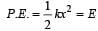At half way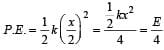31 Year NEET Previous Year Questions: Oscillations - 1 - Question 3

### In case of a forced vibration, the resonance wave becomes very sharp when the 

Detailed Solution for 31 Year NEET Previous Year Questions: Oscillations - 1 - Question 3

The resonan ce wave becomes very sharp when damping force is small.

31 Year NEET Previous Year Questions: Oscillations - 1 - Question 4

The time period of a mass suspended from a spring is T. If the spring is cut into four equal parts and the same mass is suspended from one of the parts, then the new time period will be

Detailed Solution for 31 Year NEET Previous Year Questions: Oscillations - 1 - Question 4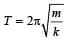When a spring is cut into n parts Spring constant for each part = nk Here, n = 4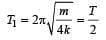31 Year NEET Previous Year Questions: Oscillations - 1 - Question 5

Which one of the following statements is true for the speed v and the acceleration a of a particle executing simple harmonic motion ?

Detailed Solution for 31 Year NEET Previous Year Questions: Oscillations - 1 - Question 5

When v is maximum, a is zero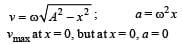31 Year NEET Previous Year Questions: Oscillations - 1 - Question 6

Two spr ings of spring constants k1 and k2 are joined in series. The effective spring constant of the combin ation is given by 

Detailed Solution for 31 Year NEET Previous Year Questions: Oscillations - 1 - Question 6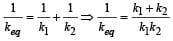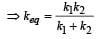31 Year NEET Previous Year Questions: Oscillations - 1 - Question 7

A particle executing simple harmonic motion of amplitude 5 cm has maximum speed of 31.4 cm/s. The frequency of its oscillation is

Detailed Solution for 31 Year NEET Previous Year Questions: Oscillations - 1 - Question 7

In S.H.M. ,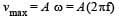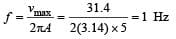31 Year NEET Previous Year Questions: Oscillations - 1 - Question 8

The potential energy of a long spring when stretched by 2cm is U. If the spring is stretched by 8 cm, the potential energy stored in it is

Detailed Solution for 31 Year NEET Previous Year Questions: Oscillations - 1 - Question 8

The potential energy of a spring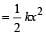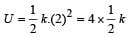For x = 8 cm,

Energy stored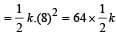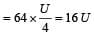31 Year NEET Previous Year Questions: Oscillations - 1 - Question 9

The phase difference between the instantaneous velocity and acceleration of a particle executing simple harmonic motion is 

Detailed Solution for 31 Year NEET Previous Year Questions: Oscillations - 1 - Question 9

Let y = A sinωt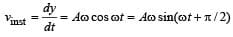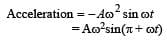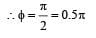31 Year NEET Previous Year Questions: Oscillations - 1 - Question 10

The particle executing simple harmonic motion has a kinetic energy K0 cos2ωt . The maximum values of the potential energy and the total energy are respectively 

Detailed Solution for 31 Year NEET Previous Year Questions: Oscillations - 1 - Question 10

We have, U + K = E where, U = potential energy, K = Kinetic energy, E = Total energy.
Also, we know that, in S.H.M., when potential energy is maximum, K.E. is zero and viceversa.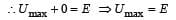Further,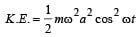But by question, 2 K .E. = K0 cosωt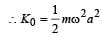Hence, total energy,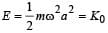∴  Umax = K0 & E = K0.

31 Year NEET Previous Year Questions: Oscillations - 1 - Question 11

A mass of 2.0 kg is put on a flat pan attached to a vertical spring fixed on the ground as shown in the figure. The mass of the spring and the pan is negligible. When pressed slightly and released the mass executes a simple harmonic motion. The spring constant is 200 N/m. What should be the minimum amplitude of the motion so that the mass gets detached from the pan (take g = 10 m/s2)?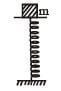Detailed Solution for 31 Year NEET Previous Year Questions: Oscillations - 1 - Question 11

Mass gets detached at the upper extreme position when pan returns to its mean position.
R = mg – mω2a = 0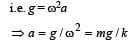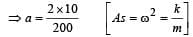⇒ a = 1/10m = 10cm

31 Year NEET Previous Year Questions: Oscillations - 1 - Question 12

A particle executes simple harmonic oscillation with an amplitude a. The period of oscillation is T. The minimum time taken by the particle to travel half of the amplitude from the equilibrium position is

Detailed Solution for 31 Year NEET Previous Year Questions: Oscillations - 1 - Question 12

Displacement from the mean position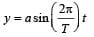According to problem y = a/2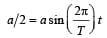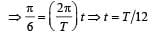This is the minimum time taken by the particle to travel half of the amplitude from the equilibrium position.

31 Year NEET Previous Year Questions: Oscillations - 1 - Question 13

Two simple harmonic motions of angular frequency 100 and 1000 rad s–1 have the same displacement amplitude. The ratio of their maximum accelerations is: 

Detailed Solution for 31 Year NEET Previous Year Questions: Oscillations - 1 - Question 13

Maximum acceleration of a particle in the simple harmonic motion is directly proportional to the square of angular frequency i.e.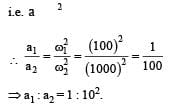31 Year NEET Previous Year Questions: Oscillations - 1 - Question 14

A point performs simple harmonic oscillation of period T and the equation of motion is given by x = a sin (ωt + π/6). After the elapse of what fraction of the time period the velocity of the point will be equal to half of its maximum velocity?



Detailed Solution for 31 Year NEET Previous Year Questions: Oscillations - 1 - Question 14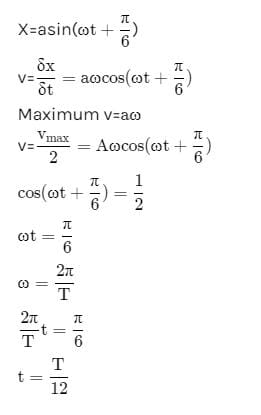31 Year NEET Previous Year Questions: Oscillations - 1 - Question 15

A simple pendulum performs simple harmonic motion about x = 0 with an amplitude a and time period T. The speed of the pendulum at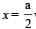will be:

Detailed Solution for 31 Year NEET Previous Year Questions: Oscillations - 1 - Question 15

Speed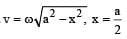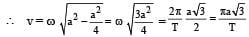31 Year NEET Previous Year Questions: Oscillations - 1 - Question 16

Which one of the following equations of motion represents simple harmonic motion? 

where k, k0, k1 and a are all postive.

Detailed Solution for 31 Year NEET Previous Year Questions: Oscillations - 1 - Question 16

a = – kX, X = x + a.
In simple harmonic motion acceleration is directly proportional to the displacement from the mean position. Also the acceleration is in the opposite direction of displacement.

31 Year NEET Previous Year Questions: Oscillations - 1 - Question 17

A block of mass M is attached to the lower end of a vertical spring. The spring is hung from a ceiling and has force constant value k. The mass is released from rest with the spring initially unstretched. The maximum extension produced in the length of the spring will be: 

Detailed Solution for 31 Year NEET Previous Year Questions: Oscillations - 1 - Question 17

If x is the maximum extension produced, we have
Mgx=1/2kx2

, on equating the decrease in the gravitational potential energy of the mass M to the increase in the elastic potential energy of the spring.

Therefore, x = 2Mg/k

31 Year NEET Previous Year Questions: Oscillations - 1 - Question 18

The displacement of a particle along the x-axis is given by x = a sin2ωt. The motion of the particle corresponds to: 

31 Year NEET Previous Year Questions: Oscillations - 1 - Question 19

The period of oscillation of a mass M suspended from a spring of negligible mass is T. If along with it another mass M is also suspended, the period of oscillation will now be 

Detailed Solution for 31 Year NEET Previous Year Questions: Oscillations - 1 - Question 19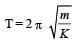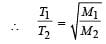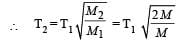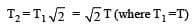31 Year NEET Previous Year Questions: Oscillations - 1 - Question 20

A par ticle of mass m is r eleased fr om rest and follows a parabolic path as shown.
Assuming that the displacement of the mass from the origin is small, which graph correctly depicts the position of the particle as a function of time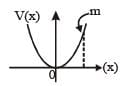Detailed Solution for 31 Year NEET Previous Year Questions: Oscillations - 1 - Question 20

The given velocity-position graph depicts that the motion of the particle is SHM.
In SHM, at t = 0, v = 0 and x = xmax
So, option (a) is correct.

31 Year NEET Previous Year Questions: Oscillations - 1 - Question 21

Out of the following functions, representing motion of a particle, which represents SHM?
(A) y = sin ωt - cosωt 
(B) y = sin3 ωt

(C)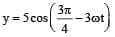(D) y = 1 + ωt + ω2t2

Detailed Solution for 31 Year NEET Previous Year Questions: Oscillations - 1 - Question 21

Only functions given in (A) & (C) represent SHM.

31 Year NEET Previous Year Questions: Oscillations - 1 - Question 22

Two particles are oscillating along two close parallel straight lines side by side, with the same frequency and amplitudes. They pass each other, moving in opposite directions when their displacement is half of the amplitude. The mean positions of the two particles lie on a straight line perpendicular to the paths of the two particles. The phase difference is [2011M]

Detailed Solution for 31 Year NEET Previous Year Questions: Oscillations - 1 - Question 22

Equation of SHM is given by x = A sin (ωt + δ) (ωt + δ) is called phase.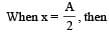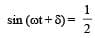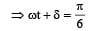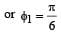For second particle,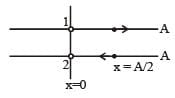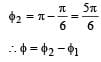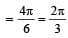31 Year NEET Previous Year Questions: Oscillations - 1 - Question 23

The damping force on an oscillator is directly proportional to the velocity. The units of the constant of proportionality are : 

Detailed Solution for 31 Year NEET Previous Year Questions: Oscillations - 1 - Question 23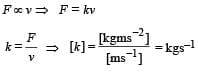31 Year NEET Previous Year Questions: Oscillations - 1 - Question 24

The equation of a simple harmonic wave is given by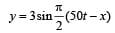Where x and y are in meters and t is in seconds.The ratio of maximum particle velocity to the wave velocity is [2012M]

Detailed Solution for 31 Year NEET Previous Year Questions: Oscillations - 1 - Question 24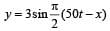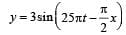on comparing with the standard wave equation

y = a sin (ωt – kx) Wave velocity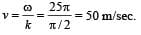The velocity of particle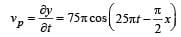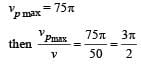31 Year NEET Previous Year Questions: Oscillations - 1 - Question 25

A particle of mass m oscillates along x-axis according to equation x = a sinωt. The nature of the graph between momentum and displacement of the particle is [NEET Kar. 2013]

Detailed Solution for 31 Year NEET Previous Year Questions: Oscillations - 1 - Question 25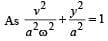This is the equation ofellipse. Hence the graph is an ellipse. P versus x graph is similar to V versus x graph.

## Physics Class 11

127 videos|464 docs|210 tests
 Use Code STAYHOME200 and get INR 200 additional OFF Use Coupon Code
Information about 31 Year NEET Previous Year Questions: Oscillations - 1 Page
In this test you can find the Exam questions for 31 Year NEET Previous Year Questions: Oscillations - 1 solved & explained in the simplest way possible. Besides giving Questions and answers for 31 Year NEET Previous Year Questions: Oscillations - 1, EduRev gives you an ample number of Online tests for practice

## Physics Class 11

127 videos|464 docs|210 tests

### How to Prepare for NEET

Read our guide to prepare for NEET which is created by Toppers & the best Teachers